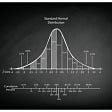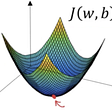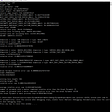# What Is the Black-Scholes Model?

Black-Scholes was the first widely used option pricing model, commonly known as Black-Scholes-Merton (BSM). The price of a European-style call option is calculated using the premise that assets such as stock shares or futures contracts will have a lognormal distribution of values following a random walk with constant drift and volatility, as well as other essential characteristics.

It accomplishes so by subtracting the stock price and the cumulative standard normal probability distribution function from the net present value (NPV) of the strike price multiplied by the cumulative standard normal distribution.

This mathematical equation calculates the potential value of derivatives and other financial instruments, taking time and other risk variables into consideration. It was created in 1973 and is currently considered one of the best methods for pricing an options contract.

# The Black-Scholes Model’s History

The Black-Scholes model was the first widely used mathematical method to calculate the theoretical value of an option contract, using current stock prices, expected dividends, the option’s strike price, expected interest rates, time to expiration, and expected volatility. It was developed in 1973 by Fischer Black, Robert Merton, and Myron Scholes. Scholes and Merton won the Nobel Memorial Prize in Economic Sciences in 1997 for their work on “a new way to determine the value of derivatives.”

# The Black-Scholes Model in Action

According to Black-Scholes, instruments such as stock shares or futures contracts will follow a random walk with steady drift and volatility, resulting in a lognormal distribution of prices. The equation calculates the price of a European-style call option based on this assumption and other significant variables.

There are five variables in the Black-Scholes equation. Volatility, the price of the underlying asset, the strike price of the option, the time until the option expires, and the risk-free interest rate are among the inputs. It is theoretically conceivable for option sellers to set rational pricing for the options they are selling using these variables.

The model also predicts that the price of highly traded assets will follow a geometric Brownian motion with steady drift and volatility. When applied to a stock option, the model takes into account the stock’s constant price movement, the time value of money, the strike price of the option, and the period until the option expires.

# Formula for the Black-Scholes Model

The formula’s equations are difficult to understand and might be daunting. To employ Black-Scholes modeling in your strategy, you don’t need to grasp or even understand the arithmetic. Options traders can use a variety of online options calculators, and many trading platforms now include extensive options analysis tools, such as indicators and spreadsheets that conduct the calculations and output the options pricing values.

Multiplying the stock price by the cumulative standard normal probability distribution function yields the Black-Scholes call option formula. The strike price’s net present value (NPV) multiplied by the cumulative standard normal distribution is then subtracted from the preceding calculation’s result.

Certain assumptions are made by the Black-Scholes model:

• During the option’s life, no dividends are paid.
• Markets are unpredictable (i.e., market movements cannot be predicted).
• Purchasing the option has no transaction fees.
• The underlying asset’s risk-free rate and volatility are known and constant.
• The underlying asset’s returns are log-normally distributed.
• The option is European and can only be exercised at expiration.

# Pitfalls of the Black-Scholes Model

As previously noted, the Black-Scholes model is exclusively used to price European options and does not account for the possibility of exercising U.S. options before the expiration date.

Furthermore, the other assumptions — that there are no transaction costs or taxes; that the risk-free interest rate is constant for all maturities; that short selling of securities with use of proceeds is allowed; and that there are no risk-free arbitrage opportunities — can result in prices that differ from those in the real world.

However, while the original Black-Scholes model did not account for dividends paid throughout the option’s life, the model is often adjusted to account for dividends by determining the underlying stock’s ex-dividend date value. Many option-selling market makers and derivatives platforms like Spin alter the model to account for the effect of options that can be exercised before expiration.

The Black-Scholes model may sound scary, but the sooner you learn this about it, the better you will be at enhancing your trading. You only need to learn the basics and Spin will take care of everything else. If you have any questions for Spin, visit our website or reach out to us on Twitter, Telegram, and Discord and we will answer!

--

--

--

## More from Spin

Spin is the first decentralized trading platform allowing for both futures and options trading built on Solana.

Love podcasts or audiobooks? Learn on the go with our new app.

## Eigenvalues and eigenvectors: a full information guide [LA4]## Data Science through the Eyes of a Mathematician## WHAT IS AN ALGORITHM AND WHAT ARE THE DIFFERENT TYPES OF ALGORITHMS## The Future Of Maths Education## Remembering Maryam Mirzakhani — Iranian Mathematician and Winner of Fields Medal## Gradient descent explained in simple way## Using Reducible Functions in Math Problems## Computationally demonstrating that the encoding of pi, phi, and e in the Great Pyramid of Giza is…## Spin

Spin is the first decentralized trading platform allowing for both futures and options trading built on Solana.

## VIBRAin Academy Live Session Recap: CeFi Vs DeFi## Announcing Winners of the Testnet Trading Competition## Telegram AMA Recap on March 11th## The State of Decentralized Derivatives Trading: Research #09